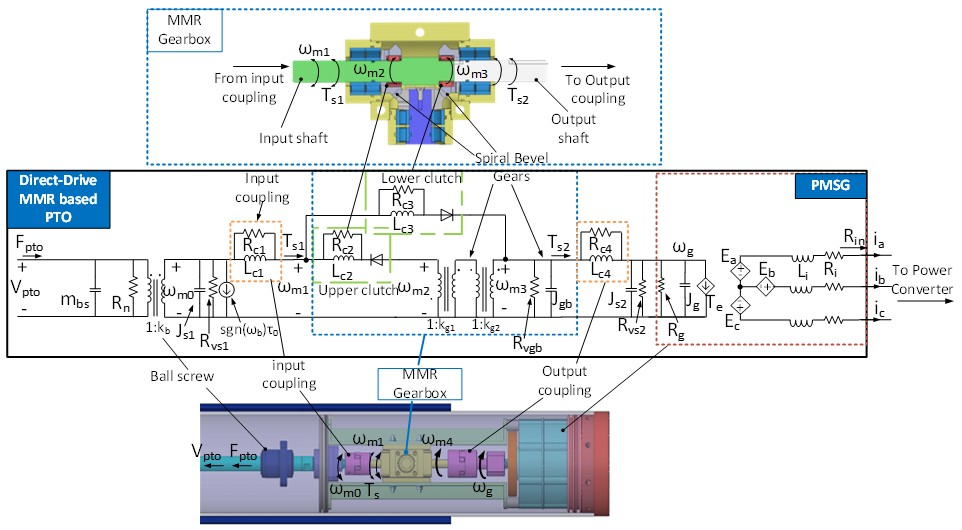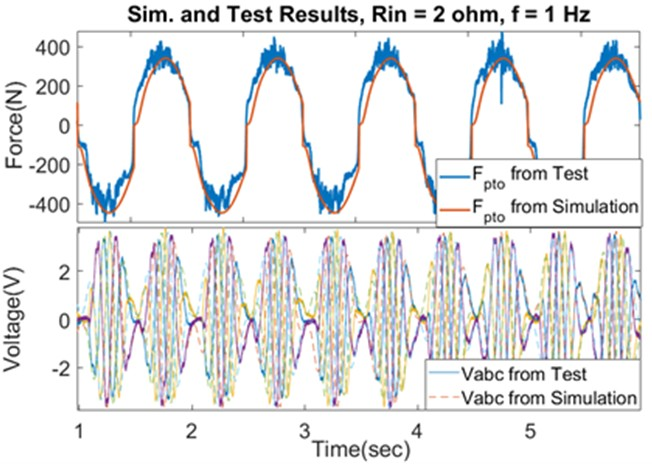Contact Us
LIBRARY

# Equivalent Circuit for Power Take-off in Wave Energy HarvestingFig. 1. Design of the PTO and its equivalent circuit including the ball-screw, MMR gearbox, mechanical couplings, shafts, and generator
This paper provides a methodology to build a high fidelity equivalent circuit for nonlinear power take-off (PTO) and simplifies the mathematical model with an equivalent circuit model. The pa-rameters in the circuit model are extracted through multiple testing conditions, and the resulting model with extracted parameters is validated under time domain with multiple test conditions. A point-absorber type wind energy conversion (WEC) system includes two parts: the floating buoy that captures the ocean wave force, and the mechanical type PTO column, shown in Fig. 1. The relative linear motion between the PTO column and the buoy will drive the ball-screw through the push tube. The ball-screw nut, embedded at the end of the push tube, will transfer the linear reciprocal motion to a bi-directional rotational motion on the input shaft of the PTO. The nonlinear mechanical motion rectifier (MMR) gearbox converts the bidirectional motion into a uni-directional rotation on the output shaft and the generator. This is accomplished through a nonlinear system identification method by comparing the results from the simulation circuit model and the experimental results. The equivalent circuit model of the overall mechanical system is built as Fig. 1. The results in Fig. 2 show that the equivalent circuit can be used to predict the mechanical input force of the nonlinear PTO time domain in the time domain, and the mechanical efficiency of the system, under various operating modes. The multiphysics circuit-based model can accurately predict the characteristics of PTO. Compared to a mathematical only model, it can simplify the modeling and parameter extraction process.Fig. 2. Time domain results of PTO input force Fpto and output voltage Vabc from the bench test and simulation
INDUSTRY PARTNERS